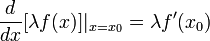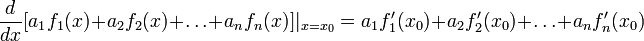# Differentiation is linear

View other differentiation rules
This article gives a statement of the form that a certain operator from a space of functions to another space of functions is a linear operator, i.e., applying the operator to the sum of two functions gives the sum of the applications to each function, and applying it to a scalar multiple of a function gives the same scalar multiple of its application to the function.

## Statement

### In terms of additivity and pulling out scalars

The following are true:

• Differentiation is additive, or derivative of sum is sum of derivatives: If$f$ and$g$ are functions that are both differentiable at$x = x_0$, we have:$\frac{d}{dx}[f(x) + g(x)]_{x = x_0} = f'(x_0) + g'(x_0)$

or equivalently, the following holds whenever the right side is defined (see concept of equality conditional to existence of one side):$(f + g)'(x_0) = f'(x_0) + g'(x_0)$

In point-free notation, we have the following whereever the right side is defined (see concept of equality conditional to existence of one side):$(f + g)' = f' + g'$

• Constants (also called scalars) can be pulled out of differentiations: If$f$ is differentiable at$x = x_0$ and$\lambda$ is a real number, then:$\frac{d}{dx}[\lambda f(x)]|_{x = x_0} = \lambda f'(x_0)$

### In terms of generalized linearity

Suppose$f_1, f_2, \dots, f_n$ are functions that are all differentiable at a point$x_0$ and$a_1, a_2, \dots, a_n$ are real numbers. Then:$\frac{d}{dx}[a_1f_1(x) + a_2f_2(x) + \dots + a_nf_n(x)]|_{x = x_0} = a_1f_1'(x_0) + a_2f_2'(x_0) + \dots + a_nf_n'(x_0)$

## Facts used

1. Limit is linear: This states that the limit of the sum is the sum of the limits and scalars can be pulled out of limits.

## Proof

We prove here the two-sided versions. Analogous proofs exist for the one-sided versions, and these use the one-sided versions of Fact (1).

Given:$f$ and$g$ are functions that are both differentiable at$x = x_0$.
To prove:$f + g$ is differentiable at$x = x_0$, and$\! (f + g)'(x_0) = f'(x_0) + g'(x_0)$
Proof: Our proof strategy is to start out by trying to compute$\! (f + g)'(x_0)$ as a difference quotient, and keep simplifying this, using Fact (1) in the process.